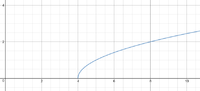Find a value(s) for x that would make this function undefined?

MarkFL

Super Moderator
Staff member
Hello, and welcome to FMH!If you graph that function, you should see something like this:Based on this, what values of $$x$$ would you say are outside of the domain?

To find this algebraically, we must observe that the radicand (expression under the radical) cannot be negative, and so to find what values of $$x$$ do make that radicand negative, we could write:

$$\displaystyle x-4<0$$

What happens if you add 4 to both sides of the inequality?

strahan

New member
Hello, and welcome to FMH!If you graph that function, you should see something like this:

View attachment 11507

Based on this, what values of $$x$$ would you say are outside of the domain?

To find this algebraically, we must observe that the radicand (expression under the radical) cannot be negative, and so to find what values of $$x$$ do make that radicand negative, we could write:

$$\displaystyle x-4<0$$

What happens if you add 4 to both sides of the inequality?
Thank you heaps for your help!

JeffM

Elite Member
With respect to the second question, can you see any values of x for which b(x) is NOT defined?

Do you see what to do next?